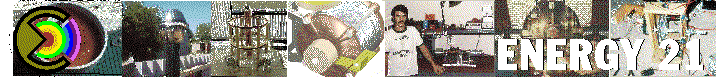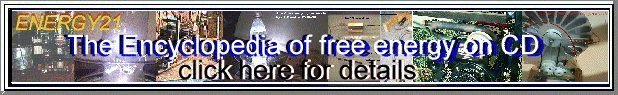## Estimating the magnitude of the Electric Field

A lot of practical high voltage design requires knowing what the maximum E-field is, for insulation design, corona reduction, etc. The exact field can, of course, be calculated numerically by solving Laplace's equation over a suitable field with appropriate boundary conditions. As complicated and time consuming as this is, it is necessary when performance is critical, in integrated circuit design, designs for absolute minimum cost, and so forth. However, for more run of the mill experimentation and use, where a little overdesign can be tolerated, approximations to the field are just as useful.

The mean or average field is just the voltage difference divided by the distance between the conductors. For the proverbial infinite flat plates, this makes the calculation simple.

Emax = Eaverage = V / Distance

#### For two concentric cylinders (i.e. like coaxial cable) the maximum field is:

Emax = V / ( Rinner * LN( Router / Rinner))

where:
Rinner is radius of the inner electrode
Router is the radius of the outer electrode
LN() is the log base e of the argument
V is the voltage between the electrodes

#### For concentric spheres, using the same variables, the maximum field is:

Emax = V * Router / (Rinner *(Router - Rinner))

#### For two parallel cylinders of equal radius:

Emax = V * SQRT(D^2 - 4 * R^2)/ (2 * R*(D-R)*INVCOSH(D/(2*R)))

approx equal to: V / (2*R) * LN(D/R) if D>>R

where:
D is distance between the centers of the conductors
R is the radius of the conductors

#### For two spheres:

Emax = approximately V/S * F

where:
S is spacing between spheres = D - 2*R
F is a field enhancement factor =

F = (S/R+1) * sqrt( (S/R+1)^2+8)/4

For spheres, if S>>R then Emax = approx V/ (2*R)

#### For other configurations:

Emax = Eaverage * F

For sphere/plane: F = 0.94*Spacing/Radius + 0.8

For cylinder/plane: F = 0.25 * Spacing / Radius + 1.0

Copyright 1998, Jim Lux / efield1.htm / 12 March 1998 / Back to HV Home / Back to home page / Mail to Jim## Main Links DirectoryWeb www.IceStuff.com Encyclopedia of free energy now on CD click for more information